Latest Banking jobs   »

# Reasoning Ability Quiz For FCI Phase I 2023- 14th January

Direction (1-3): Read the following information carefully and answer the questions which follow.
Point S is to the south of Point F. Point S is 9m to the west of Point C. Point G is 9m to the north of Point E. Point U is 8m to the south of Point C. Point G and F lie in the same straight line. Point S is the midpoint between Point C and E. Point J is 5m to the east of Point U.

Q1. What is the total distance between point G and point U?
(a) 42m
(b) 39m
(c) 44m
(d) 35m
(e) None of these

Q2. If point T is 5m west of point F, then what is the direction of point S with respect to point T?
(a) South
(b) South-east
(c) North-east
(d) North-west
(e) None of these

Q3. If point T is 5m west of point F, then which of the following points are to the north-west of Point C?
(a) Point T and Point J
(b) Point T, Point F and Point G
(c) Point F, Point T and Point U
(d) Point S and Point E
(e) None of these

Direction (4-8): Study the following data carefully and answer the questions accordingly.
‘Money calculate banks’ is written as ‘cz no jp’,
‘Increases US tension’ is written as ‘hb rt db’,
‘Banks tension US’ is written as ‘rt cz db’
‘Calculate banks US’ is written as ‘cz jp db’.

Q4. What is the possible code for ‘banks fraud’?
(a) rt ba
(b) jp tr
(c) no dc
(d) pr cz
(e) None of these

Q5. What is the code for ‘calculate money’?
(a) db no
(b) cz jp
(c) jp no
(d) cz rt
(e) Cannot be determined

Q6. What is the code for ‘US’?
(a) cz
(b) rt
(c) db
(d) jp
(e) None of these

Q7. What is the possible code for ‘towards tension increases’?
(a) rt hb jp
(b) db hb mt
(c) cz rt mp
(d) hb cd rt
(e) None of these

Q8. What is the code for ‘US increases banks’?
(a) jp cz hb
(b) db hb rt
(c) hb cz db
(d) rt cz db
(e) Cannot be determined

Direction (9-13): Study the following data carefully and answer the questions accordingly.
Eight people are sitting around a square table. Four of them are sitting at the corners facing outside and the remaining four are sitting at the sides of the table facing inside.
I sits third to the right of Q. O is a neighbor of I. O sits immediate left of W who is not a neighbor of U. Two people sit between W and S when counted right of W. S sits second to the left of Q. N is a neighbor of S but does not sit at the corner. T sits second to the right of N.

Q9. Who among the following sits third to the left of T?
(a) O
(b) I
(c) W
(d) Q
(e) None of these

Q10. How many people sit between U and O when counted right of O?
(a) Three
(b) Two
(c) One
(d) Four
(e) None

Q11. Who among the following are neighbors?
(a) U, N
(b) U and the one who sits opposite O
(c) Q and the one who sits immediate right of O
(d) N, Q
(e) None of these

Q12. Find the correct statement/statements from the following.
I. Three people sit between S and W when counted either of sides.
II. W and U face the same direction.
III. N sits third to the left of O.
(a) Only I
(b) Both I and III
(c) Both II and III
(d) Only III
(e) All are correct

Q13. Four from the following are similar in a certain way and forms a group. Find out the one who does not belong to that group.
(a) T
(b) N
(c) I
(d) W
(e) S

Q14. How many such pairs of letters are there in the word ‘POLYTHENE’ each of which has as many letters between them in the word as in the English alphabet (Both forward and backward)?
(a) One
(b) Two
(c) Three
(d) Four
(e) More than four

Q15. How many such pairs of letters are there in the word ‘FILMMAKER’ each of which has as many letters between them in the word as in the English alphabet (Both forward and backward)?
(a) One
(b) Two
(c) Three
(d) Four
(e) More than four

Solutions

Solution (1-3):
Sol.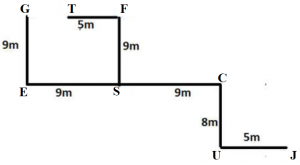S1. Ans. (d)
S2. Ans. (b)
S3. Ans. (b)

Solution (4-8):
Sol.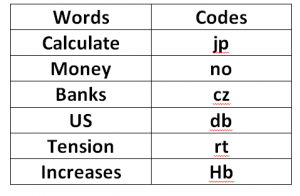S4. Ans. (d)
S5. Ans. (c)
S6. Ans. (c)
S7. Ans. (d)
S8. Ans. (c)

Solutions (9-13):
Sol.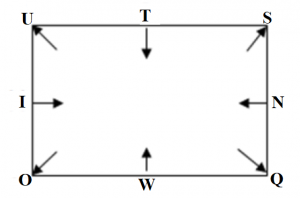S9. Ans. (d)
S10. Ans. (c)
S11. Ans. (d)
S12. Ans. (d)
S13. Ans. (e)

S14. Ans. (c)
Sol.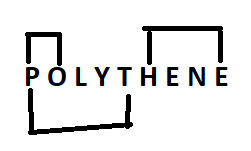S15. Ans. (e)
Sol.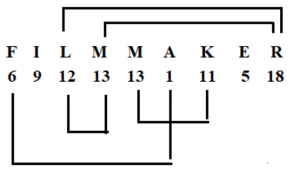## FAQs

### What is the FCI Assistant Grade 3 Phase 1 Exam Date 2023?

The FCI Assistant Grade 3 Phase 1 Exam Dates 2023 are 1st, 7th, 14th & 21st January 2023.

#### Congratulations!Union Budget 2023-24: Free PDF